# Test: Distance And Speed- 1

## 15 Questions MCQ Test Quantitative Aptitude for GMAT | Test: Distance And Speed- 1

Description
Attempt Test: Distance And Speed- 1 | 15 questions in 30 minutes | Mock test for GMAT preparation | Free important questions MCQ to study Quantitative Aptitude for GMAT for GMAT Exam | Download free PDF with solutions
QUESTION: 1

### Alice, while driving to her workplace completes an initial distance of 50 miles in 2 hours and remaining 60 miles in 3 hours. What is the average speed in miles per hour for her entire journey?

Solution:

Average speed :-

Total distance / Total time

⇒ (50+60) miles / (2+3) hours

⇒ 110/ 5

⇒ 22 miles / hour

QUESTION: 2

Solution:
QUESTION: 3

### Alan starts his journey from point A at sharp 10.00am. He drives at an average speed of 30 miles per hour and reaches Point B at 11.00 am. Bob starts his journey 15 mins after Alan started his journey and travels via same route as Alan did. If Alan and Bob both reached point B exactly at the same time, then what is Bob’s average speed (in miles per hour)?

Solution:
QUESTION: 4

A speed boat travels at a speed of x miles per hour in still water. With a favourable current, the speed boat travels downstream and reaches its destination in 2.5 hours. The speedboat travels back upstream against the current and covers the same distance in 3.5 hours.  If the speed of the current is 1mph, then what is the value of x?

Solution:
QUESTION: 5

During a trip, Charles covered the first part of a certain distance at an average speed of 30 miles per hour and the remaining part at an average speed of 50 miles per hour. If the ratio of time taken to cover the first part and remaining part is 2: 3, then what is the total distance that Charles travelled?

(1) It took a total of 5 hours to complete the entire distance.

(2) 2/7th of the entire distance was covered during the first part

Solution:
QUESTION: 6

Two dogs are running towards each other from opposite ends of a two-mile track. Dog 1 has a speed of 12 mph and Dog 2 has a speed of 8 mph. How many miles from Dog 1’s starting position will they meet?

Solution:

Step 1: Question statement and Inferences

Let the two dogs meet at a distance of x miles from the Starting position of Dog 1.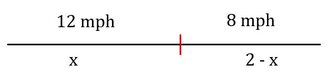So, Dog 1 covers a distance of x miles at a speed of 12 mph to reach the meeting point.

In the same time, Dog 2 covers a distance of (2-x) miles at a speed of 8 mph to reach the meeting point

Step 2: Finding required values

We know that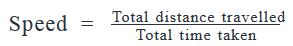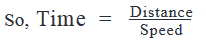Since the time taken by Dog 1 to reach the meeting point is equal to the time taken by Dog 2 to reach the meeting point, we can write: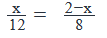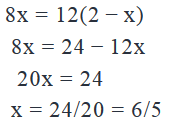The dogs will meet 6/5 miles from Dog 1’s starting position.

QUESTION: 7

Riding her bicycle downhill, Sam reached the bottom of the 10-mile trail 10 minutes faster than it took her, riding 12 miles per hour, to reach the top of the trail. What was her downhill speed?

Solution:

Step 1: Question statement and Inferences

Riding 10 miles at her new speed took Sam 10 minutes less than riding the same distance at 12 miles per hour. What was this new speed?

Step 2: Finding required values

Start by finding the time it took Sam to reach the top of the hill.

Her uphill speed, u = 12 miles per hour

Distance travelled = 10 miles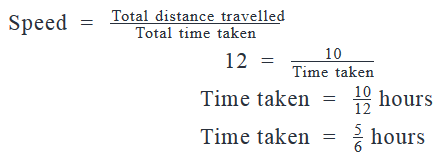Now considering the downhill journey,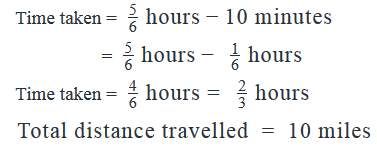Step 3: Calculating the final answer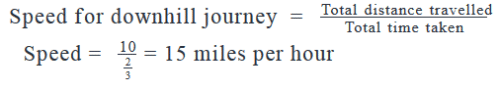QUESTION: 8

Johnny drove with an average speed of m miles per hour for h hours, increased his average speed to m + s miles per hour for an additional h hour and then increased his average speed to twice the original speed for a final h hours. Which of the following represents the distance which he drove?

Solution:

Step 1: Question statement and Inferences

Johnny drove three segments for h hours each. His speeds were m, m + s, and 2m. The question asks for the representation of the distance which he drove.

We know that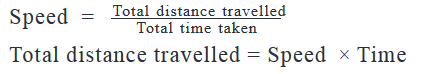Step 2: Finding required values

Multiply the time driven, h for each segment, by the speed driven for that segment, to get distances. These are hm, h(m + s), and 2hm, respectively.

Step 3: Calculating the final answer

The total distance traveled is hm + h(m + s) + 2hm, which factors out to h(4m +s).

QUESTION: 9

Emily rode x miles from her home at a speed of p miles per hour before running out of fuel.  She then walked her motorcycle at 8 meters per minute till a few miles further before she met her friend. Emily’s friend dropped her back home, driving along the same route at a rate that was 50% greater than Emily’s riding speed for x miles. If the total journey took t hours, how many miles did Emily walk her motorcycle for? (Given : 1000 meters = 0.62 miles)

Solution:

Given:

Let’s call Emily’s journey from her home till she meets her friend as her “Forward Journey” and her journey from when she meets her friend  till her home as her “Return Journey”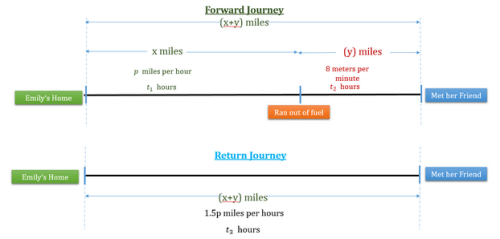Forward Journey

• Home to “Ran out of fuel” point
• Distance travelled = x miles
• Driving speed = p miles per hour
• Let the time taken be t1 hours.
• “Ran out of fuel” point till she meets her friend
• Speed of walking= 8 meters per minute
• Let the distance covered be y miles
• Let the time taken while walking = t2 hours.
• Total distance covered in Forward Journey = (x + y) miles

Return Journey

• From when Emily meets her friend to Home
• Total distance travelled = (x + y) miles
• Distance in forward journey = Distance covered in return journey
• Speed of driving = 50% more than p miles per hour
• Let the total time taken for the return journey be t3 hours.

So our DST table would look like this: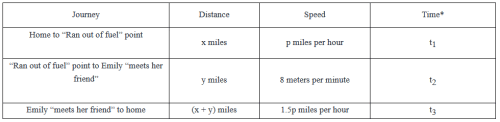*(t+ t+ t3) = t hours

To Find: How many miles she has walked her motorcycle = y = ?

1. To find the value of y, let’s focus on the Home to “Ran out of fuel” point row of the DST table.
1. To calculate the value of y from here, we need to know the speed and the time, but t2 is unknown to us.
2. We observe that t2 is also present in the equation of time (t+ t+ t3) = t hours
1. To calculate t2 we need the value of t1 and t3.
3. We observe that t3 is present in the “Emily meets her friend to home row” of the DST table.
1. From here we can express t3 in terms of x, y and p.
4. We observe that t1 is present in the Home to “Ran out of fuel” point row of the DST table.
1. From here we can express t1 in terms of x and p.
5. So we will have 4 equations and 4 variables, and thus we will be able to find the value of y.

Approach

1. From the “Emily meets her friend to home” row of the DST table, we will get t3
•  in terms of x , y and p.
• From home to “Ran out of fuel” point row of the DST table, we will get t1
•  in terms of x and p.
• From “Ran out of fuel point to Emily meets her friend” row of the DST table, we will get t2
•  in terms of y.
• Putting values of t1, t2 and t3 in the equation (t+ t+ t3) = t hours, we will be able to find the value of unknown i.e. p.

Calculation

1. From the “Emily meets her friend to home” row of the DST table, we will get t3  in terms of x , y and p.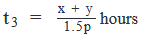2. From home to “Ran out of fuel” point row of the DST table, we will get t1 in terms of x and p.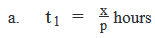3. From “Emily “meets her friend” to home  row of the DST table, we will get t2 in terms of y.

1. Speed = 8 meters per minute
1. Here speed is given to us in different units
2. Making the units consistent
3. Speed =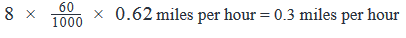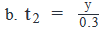4. (t+ t+ t3) = t hours

1. Putting values of t1, t2 and t3, we have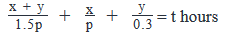• To get rid of  the variable 'p' in the denominator multiply both sides of the equation with 'p'
• So, we get :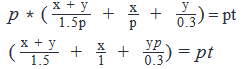To get rid of the decimal in the denominator multiply both sides of the equation by 1/10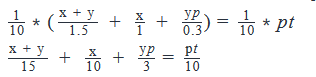• LCM (15,10, 3) = 30
• Multiplying both sides of the equation by 30, we get :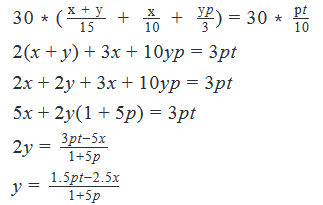QUESTION: 10

To reach her office from her home, Karen traveled x miles at a speed of y miles per hour. On her way back, via the same route, she travels for ten miles before stopping for an hour. If Karen drove at y miles per hour till she stopped, by what percentage should she increase her speed so that the overall time taken to reach back home from the office is the same as that taken to reach the office from home?

Solution:
QUESTION: 11

Jonathan drove from City A to City B at a rate of 1.2 minutes per kilometer. He then drove back to City A from City B , along the same route, at 1 minute per kilometer. If he took anywhere between 3 hours to 5 hours to travel from City A to City B and between 2 hours to 3 hours on his way back, what could be the distance between the two cities?

Solution:
QUESTION: 12

Phil drives east from his home for 2 hours before realizing that he will run out of fuel in another 70 miles. Nevertheless, he drives for another 10 miles east before returning back home via the same route. If he drives at a constant speed throughout his journey and returns home with fuel left for another 10 miles, how much time does he take for his journey eastwards?

Solution:
QUESTION: 13

Every day, Tom walks from his home to his office, via the same route, covering s feet at a speed of x feet per minute. Today he took a different route and ended up walking 10% more than he usually does, at a speed that was 100 meters per minute faster than his usual speed. What is the percentage change in the time he took today compared to the time he takes on a usual day? (1 feet =0.3 meter)

Solution:
QUESTION: 14

A train takes two hours to travel from one station to the other. What is the distance between the two stations?

(1) The train covers one third of the total distance in the first hour at an average speed of 80 mph.

(2) The average speed during the second hour is 160 mph.

Solution:
QUESTION: 15

A man rows at the rate of 5 mph in still water. If the river runs at the rate of 1 mph, it takes him 1 hour to row to a place and come back. What is the total distance covered by him in miles?

Solution:

Explanation : Speed downstream = (5 + 1) kmph = 6 mph.

Speed upstream = (5 - 1) kmph = 4 mph.

Let the required distance be x m.

Then,   x/6 + x/4 = 1

2x + 3x = 12

5x = 12

x = 2.4 m.Use Code STAYHOME200 and get INR 200 additional OFF Use Coupon Code